## Sample Problems from Chapter 14

Chapter 14: Slopes and The Derivative

1. Slope, rate and ratios.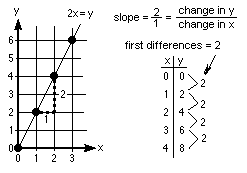2. Linear graphs and their slopes.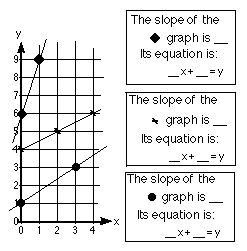3.Alec's slopes of mountains and stairs.
Use a topographic map to find the slope of your area of the country or the slope of a mountain and the slope of a ramp or the slope of the steps in your house.
4. Stories from graphs.
5. Estimating slopes: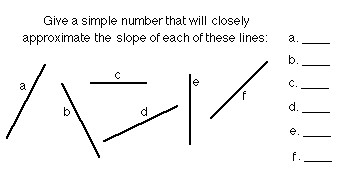6. Using the computer program Derive to zoom in on a curve at a point, then find the slope of the tangent at that point.When you zoom in at the point, the curve approaches a straight line! Increase the number grid points so you can find the slope more easily.

On the right, going down, we zoom in on the curve y = x2 at the point (1,1).

```

```
The second graph shows the same curve, again centered at (1,1), but the scale is X: 0.2, Y: 0.2 .
```

```
` `
`The third graph ends up 0.02 of a unit apart. Notice what happens. In this small portion `
`of the graph, the curve looks like a straight line. The slope of this line is essentially `
`the slope of the curve or the slope of the tangent to the curve at the point (1,1). `
`Using the dots on the screen, we can find the slope of this line, which is 2. `
`The slope of the tangent to the curve y = x2   at (1,1) is 2.`

Zoom in on other points like (2,4) and (3,9), and make a table like this:
x-coordinate     slope of tangent
1                         2
2
3

Find a rule for these pairs of numbers. Whatever you get will be the derivative of x2

```
```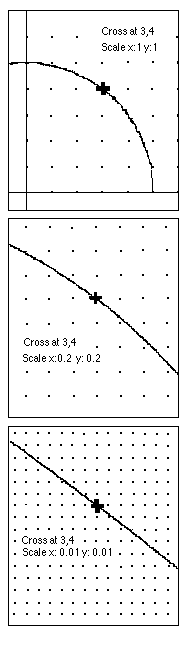On the right we zoom in on the circle x2 + y2 = 25 at the point (3,4).

```

```
Look at the third graph on the right. What's the slope of this 'line'? It looks like -3/4. The point was (3,4). What do you notice?

What would you predict would be the slope of the tangent to the circle at the point (4,3)? at (0,5)? Wow!

` `
Suppose you don't have a computer to "zoom in" on the curve x2, can you do this? SURE!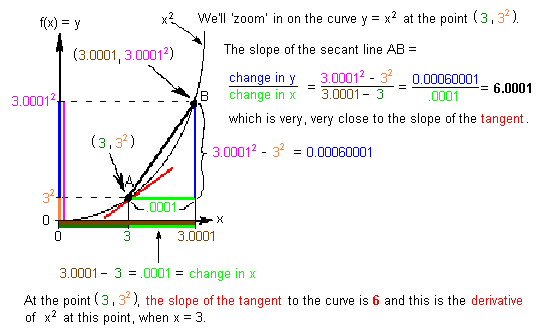Zoom in on other points like (1,2) and (2,4), and some others, and make a table like this:
x-coordinate     slope of tangent
1
2
3                         6

Find a rule for these pairs of numbers.

7. Derivatives as done in most textbooks..not done here.
8. Tickertape -- application of the derivative..not done here.
9. Now let's find the slope of the tangent to the curve y = x3 ...not done here.
10. Rectangles of constant perimeter of 20

Ways young people have solved these kinds of problems
To choose sample problems from other chapters
To order Don's materials
Mathman home